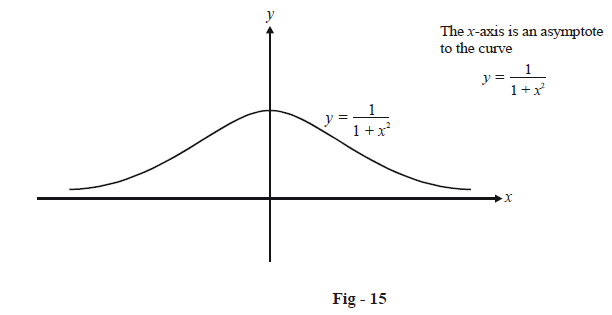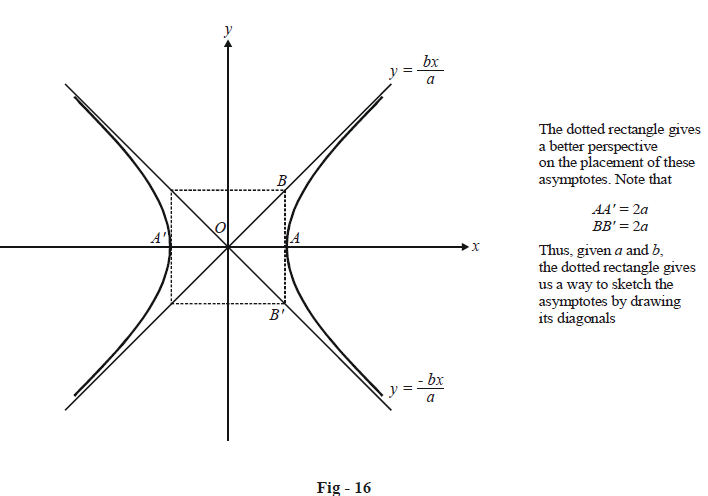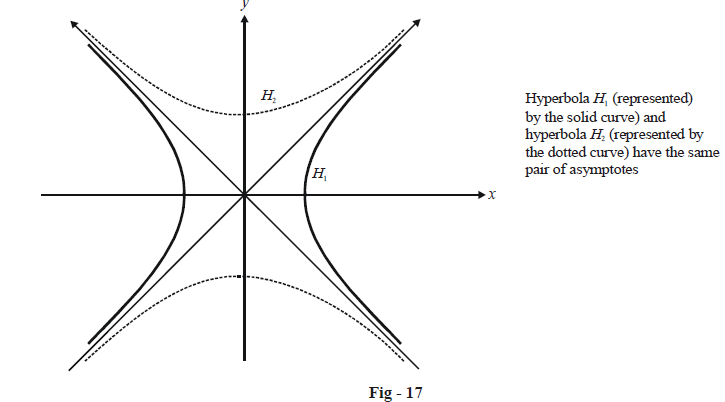# Asymptotes of Hyperbolas

Before discussing rectangular hyperbolas, we must first understand what asymptotes are.

Asymptotes : An asymptote to a curve is a straight line, to which the tangent to the curve tends as the point of contact goes to infinity.

If this sounds confusing, you can think of an asymptote as follows: an asymptote to a curve is a straight line such that the perpendicular distance of a point  $$P(x,\,y)$$   on the curve from this line tends to zero as the point P goes to infinity (along some branch of the curve).

For example, the line $$y=0$$  is an asymptote to the curve  \begin{align}y=\frac{1}{1+{{x}^{2}}}\end{align}  as shown below :Consider a hyperbola  \begin{align}\frac{{{x}^{2}}}{{{a}^{2}}}-\frac{{{y}^{2}}}{{{b}^{2}}}=1.\end{align}  Can this have asymptotes ? If so, what will be their equations ?

Let  $$y=mx+c$$ be an asymptote to the given hyperbola. We need to determine m and c.

Consider any point $$P(a\sec \theta ,\,b\tan \theta )$$  on the hyperbola. The perpendicular distance of P from our supposed asymptote  $$y=mx+c$$  will be given by

${{d}^{2}}=\frac{{{(am\sec \theta -b\tan \theta +c)}^{2}}}{1+{{m}^{2}}}\,\,\,\,\,\,\,\,\,\,\,\,\,\,\,\,\,\,\ldots \left( 1 \right)$

Now first observe that as   $$\theta \to \pm \frac{\pi }{2},$$  the point P tends to go away to infinity along some arm of the hyperbola. Thus, we want a value of m and c, such that in (1),

As\;\quad\begin{align}\theta \to \pm \frac{\pi }{2},\,\,\,\,\,d\to 0\end{align}

If you observe the expression carefully, you’ll realise that this can happen only if

$m=\pm \frac{b}{a},\,\,\,\,\,c=0$

because then the numerator will be reduced to zero. Thus, we obtain the result that the asymptotes to the hyperbola   \begin{align}\frac{{{x}^{2}}}{{{a}^{2}}}-\frac{{{y}^{2}}}{{{b}^{2}}}=1\end{align}  will be

$y=\pm \frac{b}{a}x$

$\text{or }\boxed{\frac{x}{a} \pm \frac{y}{b} = 0}$

The angle  $$\theta$$  between these asymptotes can easily be seen to be given by \begin{align}\tan \theta =\frac{2ab}{{{a}^{2}}-{{b}^{2}}}.\end{align}

The following figure should give you an idea of these asymptotes and how the hyperbola touches them at ‘infinity’:From the figure above, you might be able to infer that we can draw another hyperbola with the same pair of asymptotes, but with its transverse axis being the conjugate axis of the original hyperbola and vice-versa.H2 is called the conjugate hyperbola of H1. The transverse axis of H2 is the conjugate axis of H1 and vice-versa. It should therefore be evident that if the equation of  $${{H}_{1}}$$ is \begin{align}\frac{{{x}^{2}}}{{{a}^{2}}}-\frac{{{y}^{2}}}{{{b}^{2}}}=1,\end{align} the equation of its conjugate H2 will be

## \boxed{{H_2}:\begin{align}\frac{{{y^2}}}{{{b^2}}} - \frac{{{x^2}}}{{{a^2}}} = 1\end{align}} Conjugate hyperbola

The pair of asymptotes for both  $${H_1}$$ and  $${H_2}$$ is \begin{align}y = \pm \frac{b}{a}x\end{align}  or \begin{align}\frac{x}{a} \pm \frac{y}{b} = 0\end{align} which can be specified jointly as

$$\boxed{\frac{{{x^2}}}{{{a^2}}} - \frac{{{y^2}}}{{{b^2}}} = 0}$$  Pair of asymptotes

One very important point you must notice is that  $${H_1 }$$ and  $${H_2}$$ do not have the same eccentricity. In fact,

\begin{align} {e_{{H_1}}} = \sqrt {1 + \frac{{{b^2}}}{{{a^2}}}} \\ {e_{{H_2}}} = \sqrt {1 + \frac{{{a^2}}}{{{b^2}}}} \\ \end{align}

We can deduce another very important and useful result from this discussion : the equation of a hyperbola and the equation of its pair of asymptotes differ by just a constant. The equation of the conjugate hyperbola differs from that of the asymptotes by the same constant. This will always hold, irrespective of what coordinate system we use to write the equations. You are urged to justify logically this general result.

You will encounter the application of this result in a subsequent example.

We now return to our original topic for discussion : rectangular hyperbolas.